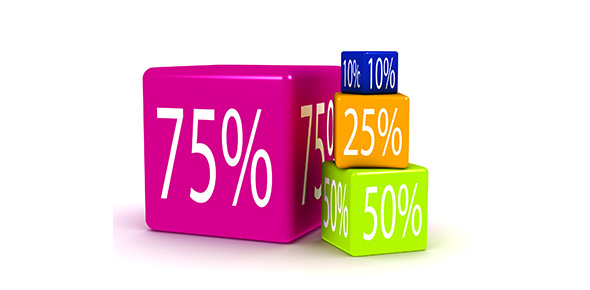Unit 7 Part I Test Review

25 Questions | Total Attempts: 95Settings• 1.
Solve with a proportion.  Round to the nearest tenth if necessary. 4 is what percent of 16?
• A.

25%

• B.

16%

• C.

40%

• D.

64%

• 2.
Solve with a proportion. Round to the nearest tenth if necessary. What percent of 70 is 5?
• A.

5%

• B.

7.1%

• C.

14%

• D.

3.5%

• 3.
Solve with a proportion. Round to the nearest tenth if necessary. What is 12.3% of 87?
• A.

707.3

• B.

10.44

• C.

1,070.1

• D.

10.7

• 4.
Solve with a proportion. Round to the nearest tenth if necessary. Find 98% of 400.
• A.

408.1

• B.

39,200

• C.

392

• D.

375

• 5.
Solve with a proportion. Round to the nearest tenth if necessary. 175 is 19% of what number?
• A.

921.1

• B.

33.3

• C.

3,325

• D.

10.9

• 6.
Solve with a proportion. Round to the nearest tenth if necessary. Find 80% of 75.
• A.

60

• B.

56.3

• C.

93.8

• D.

30

• 7.
Solve with a proportion. Round to the nearest tenth if necessary. What is 160% of 76?
• A.

12.2

• B.

121.6

• C.

152.0

• D.

47.5

• 8.
Solve with a proportion. Round to the nearest tenth if necessary. What percent of 4,000 is 12?
• A.

33.3%

• B.

30%

• C.

0.3%

• D.

48%

• 9.
Solve with a proportion. Round to the nearest tenth if necessary. 100 is what percent of 200?
• A.

50%

• B.

100%

• C.

0.5%

• D.

200%

• 10.
Solve with a proportion. Round to the nearest tenth if necessary. 420 is 17.5% of what number?
• A.

0.04

• B.

24

• C.

2,400

• D.

73.5

• 11.
Find each percent of change. Round to the nearest tenth if necessary.  State whether the percent of change is an increase or decrease. Original:  32 New:  42
• A.

31.3% decrease

• B.

23.8% increase

• C.

23.8% decrease

• D.

31.3% increase

• 12.
Find each percent of change. Round to the nearest tenth if necessary. State whether the percent of change is an increase or decrease. Original: 74 New: 124
• A.

67.6% decrease

• B.

40.3% decrease

• C.

67.6% increase

• D.

40.3% increase

• 13.
Find each percent of change. Round to the nearest tenth if necessary. State whether the percent of change is an increase or decrease. Original: 27 New: 18
• A.

33.3% increase

• B.

50% decrease

• C.

50% increase

• D.

33.3% decrease

• 14.
Find each percent of change. Round to the nearest tenth if necessary. State whether the percent of change is an increase or decrease. Original: 141 New: 96
• A.

31.9% decrease

• B.

46.9% decrease

• C.

31.9% increase

• D.

46.9% increase

• 15.
Find the simple interest to the nearest cent. \$150 at 7.5% for 3 years.
• A.

\$3.38

• B.

\$3,375.00

• C.

\$33.75

• D.

\$337.50

• 16.
Find the simple interest to the nearest cent. \$4,300 at 2.25% for 3 ¼ years.
• A.

\$31,443.75

• B.

\$322.18

• C.

\$314.44

• D.

\$3,221.78

• 17.
Find the simple interest to the nearest cent. \$2,075 at 12.5% for 18 months.
• A.

\$415.00

• B.

\$4,668.75

• C.

\$389.06

• D.

\$38,906.25

• 18.
Find the simple interest to the nearest cent. Jose placed \$505 in a savings account at a simple interest rate of 4.5%.  How much interest will the account earn in 4 years?
• A.

\$909.00

• B.

\$90.90

• C.

\$99.00

• D.

\$9,090.00

• 19.
Find the simple interest to the nearest cent. If Margo placed \$1,014 in a savings account for 2 years, find the interest she would earn if the simple interest rate is 3.75%.
• A.

\$7,605.00

• B.

\$76.05

• C.

\$76,050.00

• D.

\$760.50

• 20.
Complete each conversion.  Round to the nearest hundredth. 2 miles = _______ feet
• A.

10,560

• B.

5,280

• C.

1.61

• D.

3.22

• 21.
Complete each conversion. Round to the nearest hundredth. 5 gallons = _______ quarts
• A.

2.5

• B.

4

• C.

20

• D.

25

• 22.
Complete each conversion. Round to the nearest hundredth. 72.6 cm = _______ mm
• A.

0.73

• B.

726

• C.

7,260

• D.

7.26

• 23.
Complete each conversion. Round to the nearest hundredth. 5 inches = _______ cm
• A.

12.7

• B.

1.97

• C.

19.7

• D.

127

• 24.
Complete each conversion. Round to the nearest hundredth. 2,000 lbs = _______ kg
• A.

4,405.29

• B.

9,080

• C.

405

• D.

908

• 25.
Complete each conversion. Round to the nearest hundredth. 70 miles/hour = _______ meters/second
• A.

3,600

• B.

60

• C.

31.3

• D.

1.88

Related TopicsBack to top